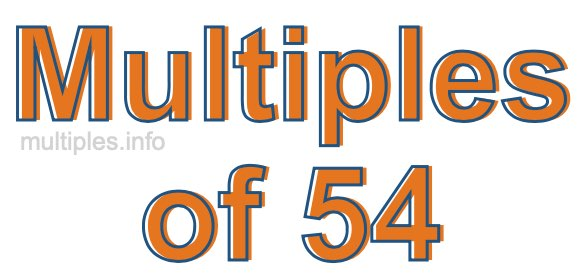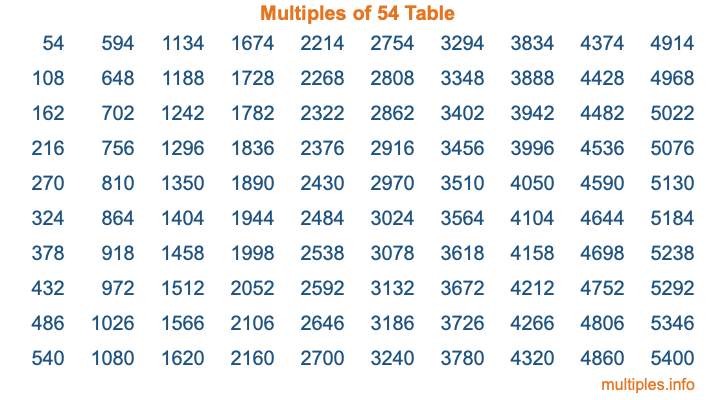Multiples of 54Welcome to the Multiples of 54 page. Here we will first teach you everything you will ever need to know about the multiples of 54, and then give you a study guide summary of everything we taught you to make sure you remember it all. Use this page to look up facts and learn information about the multiples of 54. This page will make you a multiples of fifty-four expert!

Definition of Multiples of 54
Multiples of 54 are all the numbers that when divided by 54 equal an integer. Each of the multiples of 54 are called a multiple. A multiple of 54 is created by multiplying 54 by an integer.

Therefore, to create a list of multiples of 54, you start with 1 multiplied by 54, then 2 multiplied by 54, then 3 multiplied by 54, and so on for as long as you want. Thus, the list of the first five multiples of 54 is 54, 108, 162, 216, and 270. To see a larger list of multiples of 54, see the printable image of Multiples of 54 further down on this page. We also have a category where you can choose any nth multiple of 54.

Multiples of 54 Checker
The Multiples of 54 Checker below checks to see if any number of your choice is a multiple of 54. In other words, it checks to see if there is any number (integer) that when multiplied by 54 will equal your number. To do that, we divide your number by 54. If the the quotient is an integer, then your number is a multiple of 54.

Is  a multiple of 54?

Least Common Multiple of 54 and ...
A Least Common Multiple (LCM) is the lowest multiple that two or more numbers have in common. This is also called the smallest common multiple or lowest common multiple and is useful to know when you are adding our subtracting fractions. Enter one or more numbers below (54 is already entered) to find the LCM.

Check out our LCM Calculator if you need more details about the Least Common Multiple or if you need the LCM for different numbers for adding and subtraction fractions.

nth Multiple of 54
As we stated above, 54 is the first multiple of 54, 108 is the second multiple of 54, 162 is the third multiple of 54, and so on. Enter a number below to find the nth multiple of 54.

th multiple of 54

Multiples of 54 vs Factors of 54
54 is a multiple of 54 and a factor of 54, but that is where the similarities end. All postive multiples of 54 are 54 or greater than 54. All positive factors of 54 are 54 or less than 54.

Below is the beginning list of multiples of 54 and the factors of 54 so you can compare:

Multiples of 54: 54, 108, 162, 216, 270, etc.

Factors of 54: 1, 2, 3, 6, 9, 18, 27, 54

As you can see, the multiples of 54 are all the numbers that you can divide by 54 to get a whole number. The factors of 54, on the other hand, are all the whole numbers that you can multiply by another whole number to get 54.

It's also interesting to note that if a number (x) is a factor of 54, then 54 will also be a multiple of that number (x).

Multiples of 54 vs Divisors of 54
The divisors of 54 are all the integers that 54 can be divided by evenly. Below is a list of the divisors of 54.

Divisors of 54: 1, 2, 3, 6, 9, 18, 27, 54

The interesting thing to note here is that if you take any multiple of 54 and divide it by a divisor of 54, you will see that the quotient is an integer.

Multiples of 54 Table
Below is an image of the first 100 multiples of 54 in a table. The table is in chronological order, column by column. The first column has the first ten multiples of 54, the second column has the next ten multiples of 54, and so on.The Multiples of 54 Table is also referred to as the 54 Times Table or Times Table of 54. You are welcome to print out our table for your studies.

Negative Multiples of 54
Although not often discussed or needed in math, it is worth mentioning that you can make a list of negative multiples of 54 by multiplying 54 by -1, then by -2, then by -3, and so on, to get the following list of negative multiples of 54:

-54, -108, -162, -216, -270, etc.

Multiples of 54 Summary
Below is a summary of important Multiples of 54 facts that we have discussed on this page. To retain the knowledge on this page, we recommend that you read through the summary and explain to yourself or a study partner why they hold true.

There are an infinite number of multiples of 54.

A multiple of 54 divided by 54 will equal a whole number.

54 divided by a factor of 54 equals a divisor of 54.

The nth multiple of 54 is n times 54.

The largest factor of 54 is equal to the first positive multiple of 54.

54 is a multiple of every factor of 54.

54 is a multiple of 54.

A multiple of 54 divided by a divisor of 54 equals an integer.

54 divided by a divisor of 54 equals a factor of 54.

Any integer times 54 will equal a multiple of 54.

Multiples of a Number
Here you can get the multiples of another number, all with the same attention to detail as we did for multiples of 54 on this page.

Multiples of
Multiples of 55
Did you find our page about multiples of fifty-four educational? Do you want more knowledge? Check out the multiples of the next number on our list!